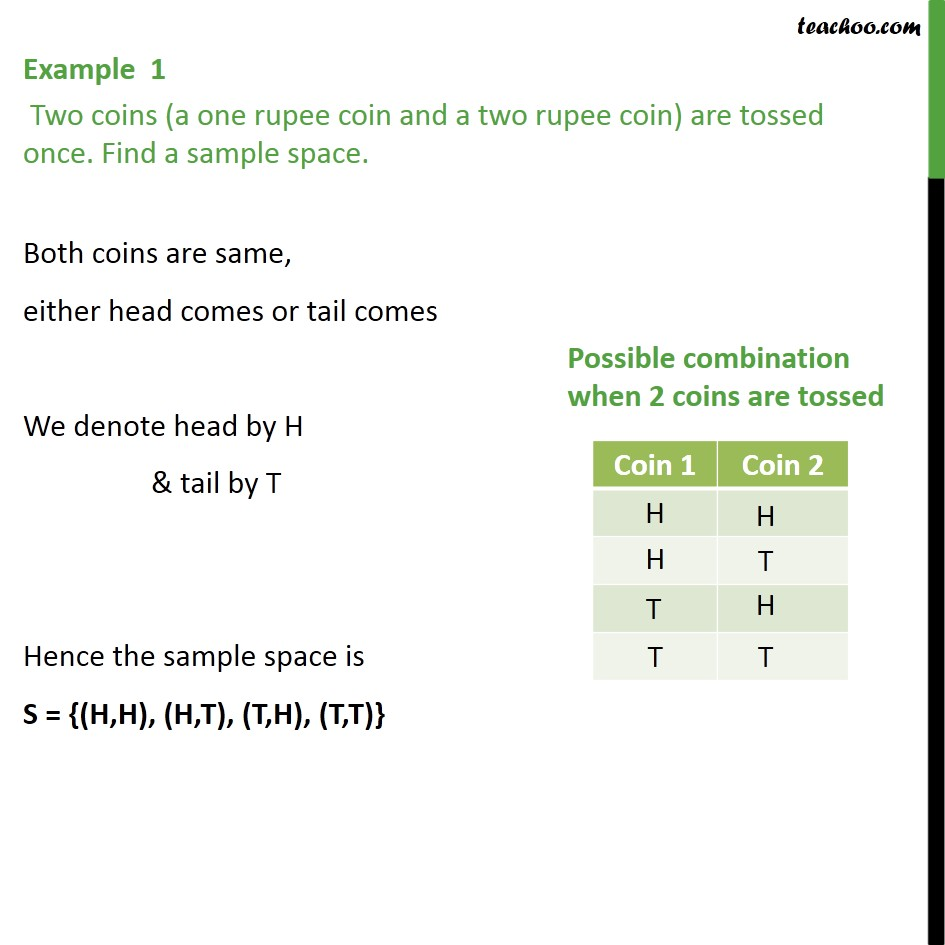1. Chapter 16 Class 11 Probability (Term 2)
2. Concept wise
3. Sample Space

Transcript

Example 1 Two coins (a one rupee coin and a two rupee coin) are tossed once. Find a sample space. Both coins are same, either head comes or tail comes We denote head by H & tail by T Hence the sample space is S = {(H,H), (H,T), (T,H), (T,T)}